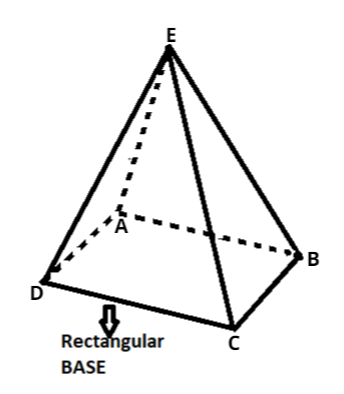Courses
Courses for Kids
Free study material
Free LIVE classes
MoreLIVE
Join Vedantu’s FREE Mastercalss

# Fill in the blanks:A rectangular pyramid has $\_\_\_$ faces.Verified
361.2k+ views
Hint:- Draw figure of rectangular pyramid.As we can see from the above figure that the base of the figure is rectangular.
And all the vertices of the base meets at a particular point.
So, this figure will be of a rectangular pyramid.
As we all know that face is any flat surface in a figure.
And, the face is a 2D shape that makes up one surface of a 3D shape.
And, edges are where two flat surfaces meet in a line segment.
And, the point where two edges come and meet up is known as the vertex of the shape.
So, in above figure there will be 5 faces and that were,
$\Rightarrow$Faces are ABCD, AEB, BEC, CED and DEA.
So, in the above figure there are 4 triangular faces and 1 rectangular face.
And, there are 8 edges in the rectangular pyramid. And that were,
$\Rightarrow$Edges are AB, BC. CD, DA, EA, EB, EC and ED.
And there are 5 vertices in the rectangular pyramid. And that were,
$\Rightarrow$Vertices are A, B, C, D and E.
Hence, the rectangular pyramid has 5 faces.
Note:- Whenever we came up with this type of question then easiest and efficient
way to find number of faces, edges or vertices in any shape is to draw the figure
that shape and then remember that face is any flat 2D surface that makes up any 3D figure.
Last updated date: 16th Sep 2023
Total views: 361.2k
Views today: 10.61k